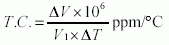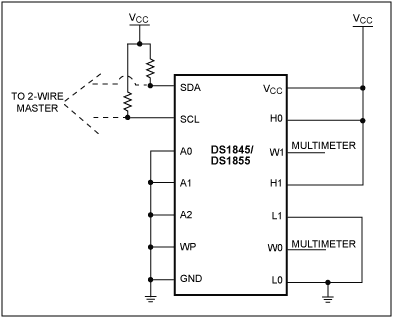# DS1845/DS1855 Temperature Coefficient Analysis

### Abstract

The temperature characteristics of digital potentiometers differ from those of traditional mechanical potentiometers. The effects of temperature on the absolute resistance of the potentiometer must be taken into consideration when designing with these types of devices as a digital potentiometer has an absolute resistance, which might vary on an order of magnitude of 10% over temperature. Other considerations, especially the resistance of the \"wiper\" which is in actuality a transmission gate, must also be taken into consideration when designing with these parts. An overview of the stability of a design in different configurations is given. The effects if wiper resistance are also explained.

Characteristic data is given for these devices in both types of configuration. More recent devices, such as the DS1847, DS1848, and others, have been designed which have mechanisms internally to compensate for these changes. Consult with a Maxim FAE for the latest technology when considering a design with these types of devices as newer, improved versions are always in development.

### Introduction

The DS1845 and DS1855 are dual nonvolatile (NV) potentiometers with memory. Both devices have two linear taper potentiometers. One potentiometer has 100 positions, and the second has 256 positions. The DS1845/1855 can operate from a 3V or 5V supply over a temperature range of -40°C to +85°C. A more in-depth analysis of the temperature coefficient is needed due to the different configurations possible with the potentiometers. Each configuration produces a different temperature coefficient. This application note discusses the temperature coefficient of the DS1845/DS1855 in the voltage divider and variable resistor configurations.

### Temperature Coefficient

The temperature coefficient changes depending on the device configuration. The data sheet states the end-to-end temperature coefficient is 750ppm/°C. In voltage divider mode, the temperature coefficient is actually much lower. The temperature coefficient is lower in voltage divider mode because the temperature coefficients of each resistor branch (R1 and R2) will cancel out each other (see Figure 1). The resistance of the wiper (RW) will then primarily affect the temperature coefficient. The equations used to calculate the temperature coefficient for each mode are shown in Equations 1 and 2.Figure 1. Resistor branches in voltage divider mode.

### Voltage Divider Mode

To measure the temperature coefficient in voltage divider mode, the H terminal was tied to VCC and the L terminal was tied to ground. Using the 2-wire interface, the potentiometer position was changed and voltage was measured on the W terminal for each potentiometer position over temperature. See Figure 2 for a drawing of the DS1845/1855 in voltage divider mode. The equation used to calculate the temperature coefficient is below.Equation 1.

Where,
ΔV = the difference between the voltage (V2 - V1) at temperatures T2 and T1 (T2 > T1), respectively
V1 = the voltage at T1
ΔT = T2 - T1Figure 2. Voltage divider mode.

### Variable Resistor Mode

To measure the temperature coefficient in variable resistor mode, the H and W terminals were tied together. The L terminal was grounded. A 1µA current was forced on the H and W terminals with the compliance set equal to VCC. Using the 2-wire interface, the potentiometer position was changed and the voltage was measured from W to ground. This measurement was repeated over temperature. Forcing a current and measuring a voltage ensures the biasing of the resistor terminal remains between VCC and ground. This ensures that the device parasitics do not affect the measurement. See Figure 3 for a diagram of the DS1845/1855 in variable resistor mode. The equation used to calculate the temperature coefficient is below.Equation 2.

Where,
ΔR = the difference between the resistance (R2 - R1) at temperatures T2 and T1 (T2 > T1), respectively
R1 = the resistance at T1
ΔT = T2 - T1Figure 3. Variable resistor mode.

Note: Tying W to L produces the same effect.

### Data

Note: The results were taken on a small sample size and are not to be considered a full characterization.

### Voltage Divider Mode

The temperature coefficient was calculated as approximately 10ppm/°C using Equation 1.Figure 4. DS1855 in voltage divider mode (pot 1).

Figure 4 shows the voltage variation of the DS1855 in voltage divider mode over temperature. VCC was set to 5V; therefore at a 50% pot setting the voltage should be 2.5V.

The calculation below shows how the temperature coefficient for Figure 4 was calculated.### Variable Resistor Mode

The temperature coefficient was calculated to be approximately 750ppm/°C using Equation 2.Figure 5. DS1845 in variable resistor mode (pot 0).

Figure 5 shows the change in resistance of the DS1845 in variable resistor mode over temperature. Pot 0 is a 10kΩ resistor; therefore at a 50% pot setting the resistance should be 5kΩ.

The calculation below shows how the temperature coefficient for the DS1845-050 in Figure 5 was calculated.Figure 6. DS1845 in variable resistor mode (pot 1).

Figure 6 shows how the resistance changes as the pot position changes. The -100 has a 100kΩ resistor, the -050 has a 50kΩ resistor, and the -010 has a 10kΩ resistor for pot 1.

### Conclusion

These results show that the temperature coefficient varies depending on the mode of the device. The results for the DS1845 and DS1855 were similar. In voltage divider mode the temperature coefficient will be on the order of 10ppm/°C. If the device is configured in variable resistor mode, then the temperature coefficient will be on the order of 750ppm/°C. If an application requires a variable resistor with a better temperature coefficient performance in variable resistor mode, then the DS1847 or DS1848 may provide a better solution. The DS1847 and DS1848 data sheets and app notes are located at www.maximintegrated.com.